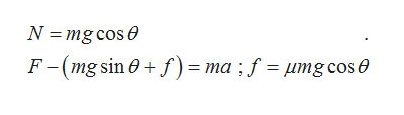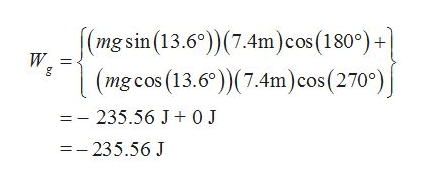# A 13.8 kg crate is pulled up a rough inclinewith an initial speed of 1.5 m/s. The pullingforce is 123.0 N parallel to the incline, whichmakes an angle of 13.6◦ with the horizontal.The coefficient of kinetic friction is 0.33 andthe crate is pulled a distance of 7.8 m.The acceleration of gravity is 9.81 m/s^2a) Find the work done by Earth’s gravityon the crate.Answer in units of J.b) Find the work done by the force of frictionon the crate.Answer in units of J.c) Find the work done by the puller on thecrate.Answer in units of J.d) Find the change in kinetic energy of thecrate.Answer in units of J.e) Find the speed of the crate after it is pulled7.8 m.Answer in units of m/s.

Question
14 views

A 13.8 kg crate is pulled up a rough incline
with an initial speed of 1.5 m/s. The pulling
force is 123.0 N parallel to the incline, which
makes an angle of 13.6◦ with the horizontal.

The coefficient of kinetic friction is 0.33 and
the crate is pulled a distance of 7.8 m.
The acceleration of gravity is 9.81 m/s^2

a) Find the work done by Earth’s gravity
on the crate.

b) Find the work done by the force of friction
on the crate.

c) Find the work done by the puller on the
crate.

d) Find the change in kinetic energy of the
crate.

e) Find the speed of the crate after it is pulled
7.8 m.

check_circle

Step 1

Let the mass of the crate be denoted by m, the pulling force as F, the force of friction as f , v the initial velocity of the block, a the acceleration of the block, N as the normal reaction of the block and μ as the coefficient of Kinetic friction. Let d denote the distance moved by the crate.

The free body diagram of the arrangement is shown below,

Step 2

From the free body diagram illustrated above, the following equations for the motion of the crate upwards the crate can be deduced.help_outlineImage TranscriptioncloseN = mg cos e F-(mg sin 0 + f )= ma ; f = umg cos 0 fullscreen
Step 3

Work done by the force of gravity is calculated as by breaking the gr...help_outlineImage Transcriptionclose|(mg sin (13.6°))(7.4m)cos(180°)+ W. (mg cos (13.6°))(7.4m)cos(270°)| =- 235.56 J+ 0 J =- 235.56 J bg fullscreen

### Want to see the full answer?

See Solution

#### Want to see this answer and more?

Solutions are written by subject experts who are available 24/7. Questions are typically answered within 1 hour.*

See Solution
*Response times may vary by subject and question.
Tagged in

### Work,Power and Energy